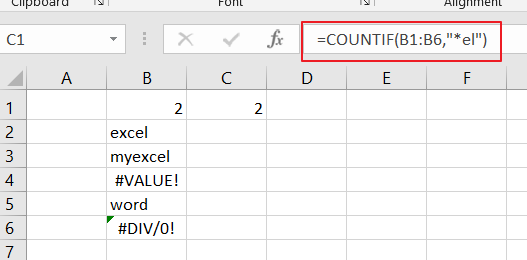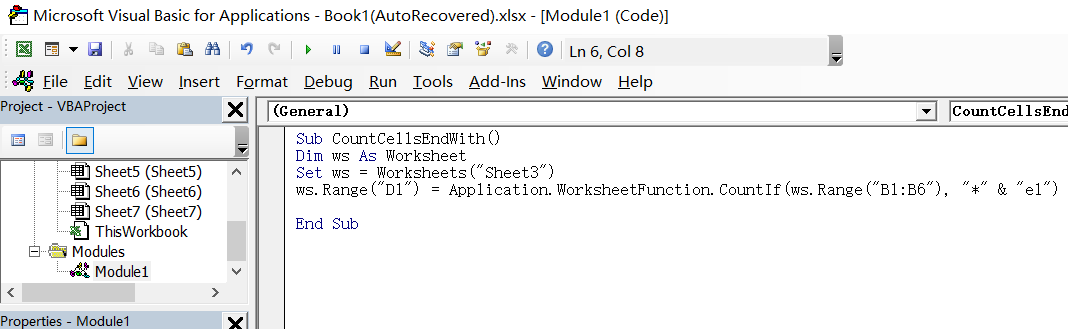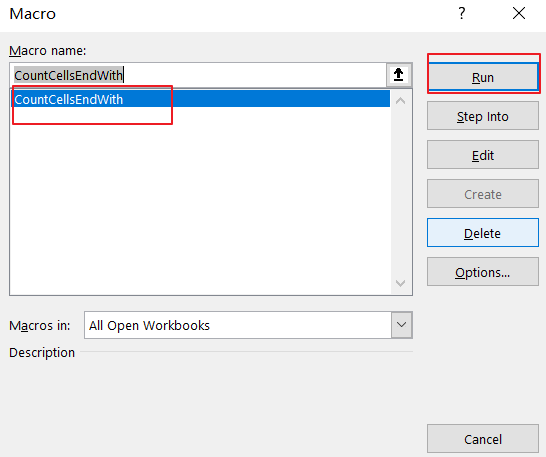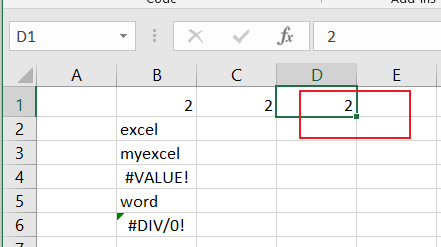# How to Count Cells that End with Specific Text in Excel

This post will guide you how to count the number of cells that end with a specific value within a range of cells using a formula in Excel 2013/2016 or Excel office 365. How do I count cells that end with a specific text through the use of VBA Macro in Excel.

## Count Number of Cells that End with a Specific Text Through Formula

If you want to count the number of cells that end with a specific text value “el” in range B1:B6, and you can use the COUNTIF function to build a formula. And you need to use the asterisk(*) sign to count the number of cells that end with “el”. Just using the following formula:

=COUNTIF(B1:B6,”*ex”)LET’S SEE THAT HOW THIS FORMULA WORKS:

The COUNTIF function can be used to count the number of cells in the range that end with a specific text by matching the content of each cell against the pattern. And the asterisk sign is a wildcard that means “match any number of characters”.

## Count Number of Cells that End with a Specific Text Through VBA

You can also use an VBA Macro to count cells if ends with a specific value in Excel. Just do the following steps:

Step1# open your excel workbook and then click on “Visual Basic” command under DEVELOPER Tab, or just press “ALT+F11” shortcut.Step2#  then the “Visual Basic Editor” window will appear.

Step3# click “Insert” ->”Module” to create a new module.Step4# paste the below VBA code into the code window. Then clicking “Save” button.```Sub CountCellsEndWith()
Dim ws As Worksheet
Set ws = Worksheets("Sheet3")
ws.Range("D1") = Application.WorksheetFunction.CountIf(ws.Range("B1:B6"), "*" & "el")

End Sub```

Note: you need to change the output range D1 to place the final result. And change the data range that you want to test the criteria against by changing the cell reference. You also need to change “el” pattern as you need .

Step5# back to the current worksheet, click on `Macros` button under `Code` group. then click `Run` button.Step6# let’s see the result:### Related Functions

• Excel COUNTIF function
The Excel COUNTIF function will count the number of cells in a range that meet a given criteria. This function can be used to count the different kinds of cells with number, date, text values, blank, non-blanks, or containing specific characters. etc. = COUNTIF (range, criteria) …